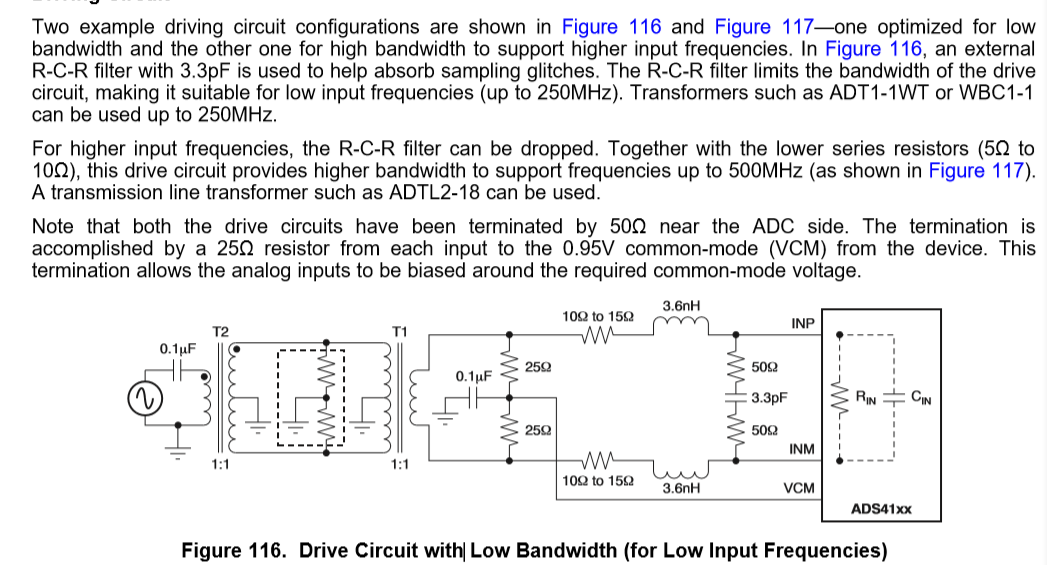If you have a related question, please click the "Ask a related question" button in the top right corner. The newly created question will be automatically linked to this question.

• TI Thinks Resolved

# ADS4129: How to calculate the filter's capacitance of the ADS4129's input

Hello!

How to calculate the filter's capacitance of the ADS4129's input!Thanks

• User,

You should be able to figure this out using Figures 114 and 115 in the data sheet. These show the resistance value of Rin and the capacitance value of Cin over frequency.

Regards,

Jim

• In reply to jim s:

Hello！

In the input frequency is 250 MHZ, the input capacitance is about 3.6 PF, the input resistance is about 500 Ω，Can you tell me the relationship between the capacitance of r-c-r and the maximum input frequency？

Thanks

User,

The r-c-r is a 250MHz low pass filter. If your IF is going to be above this frequency, remove these parts from the EVM.

Regards,

Jim

• In reply to jim s:

Hello，

I want to  set the frequency 50MHz ,How should I calculate?

Thanks!